# ISEE Upper Level Quantitative : How to find the area of a sector

## Example Questions

### Example Question #1 : How To Find The Area Of A Sector

Circle A has twice the radius of Circle B. Which is the greater quantity?

(a) The area of asector of Circle A

(b) The area of Circle B

(a) and (b) are equal.

(b) is greater.

It is impossible to tell from the information given.

(a) is greater.

(a) and (b) are equal.

Explanation:

Letbe the radius of Circle B. The radius of Circle A is therefore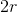.sector of a circle comprises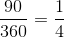of the circle. Thesector of circle A has area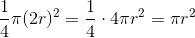, the area of Circle B. The two quantities are equal.

### Example Question #2 : Sectors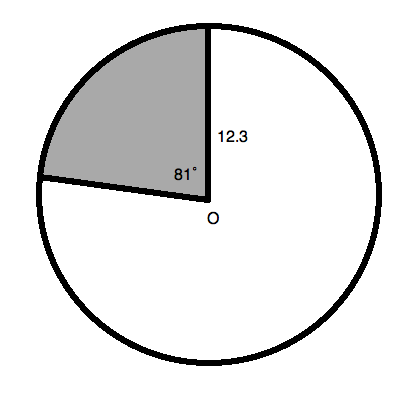What is the area, rounded to the nearest hundredth, of the sector shaded in circle O in the diagram above?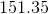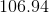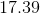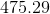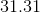Explanation:

To find the area of a sector, you need to find a percentage of the total area of the circle.  You do this by dividing the sector angle by the total number of degrees in a full circle (i.e.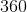˚).  Thus, for our circle, which has a sector with an angle of˚, we have a percentage of: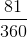Now, we will multiply this by the total area of the circle.  Recall that we find such an area according to the equation: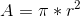For our problem,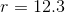Therefore, our equation is: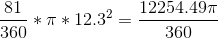Using your calculator, you can determine that this is approximately.

### Example Question #3 : Sectors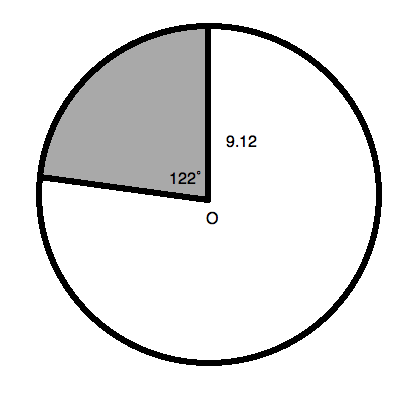What is the area, rounded to the nearest hundredth, of the sector shaded in circle O in the diagram above?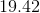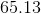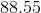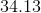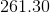Explanation:

To find the area of a sector, you need to find a percentage of the total area of the circle.  You do this by dividing the sector angle by the total number of degrees in a full circle (i.e.˚).  Thus, for our circle, which has a sector with an angle of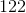˚, we have a percentage of: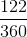Now, we will multiply this by the total area of the circle.  Recall that we find such an area according to the equation:For our problem,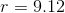Therefore, our equation is: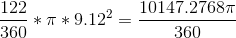Using your calculator, you can determine that this is approximately.

### Example Question #4 : Sectors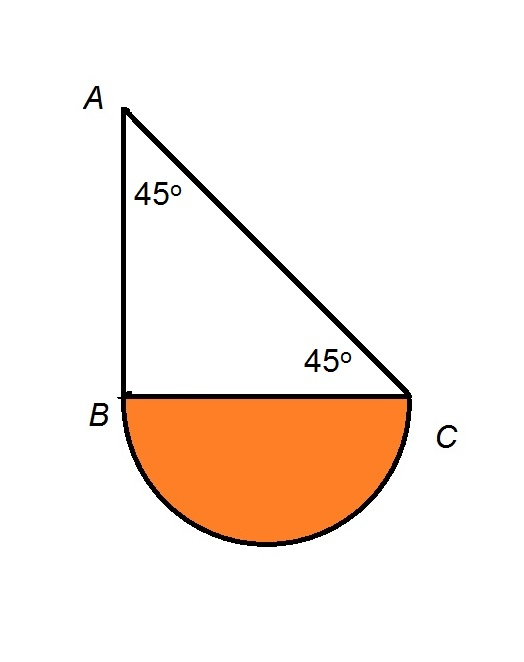Refer to the above figure, Which is the greater quantity?

(a) The area of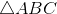(b) The area of the orange semicircle

(a) and (b) are equal

(a) is the greater quantity

It is impossible to determine which is greater from the information given

(b) is the greater quantity

(a) is the greater quantity

Explanation:has two angles of degree measure 45; the third angle must measure 90 degrees, makinga right triangle.

For the sake of simplicity, let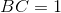; the reasoning is independent of the actual length. The legs of a 45-45-90 triangle are congruent, so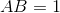. The area of a right triangle is half the product of its legs, so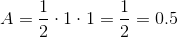Also, if, then the orange semicircle has diameter 1 and radius. Its area can be found by substituting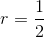in the formula: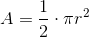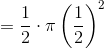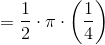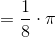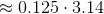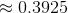has a greater area than the orange semicircle.

### Example Question #5 : Sectors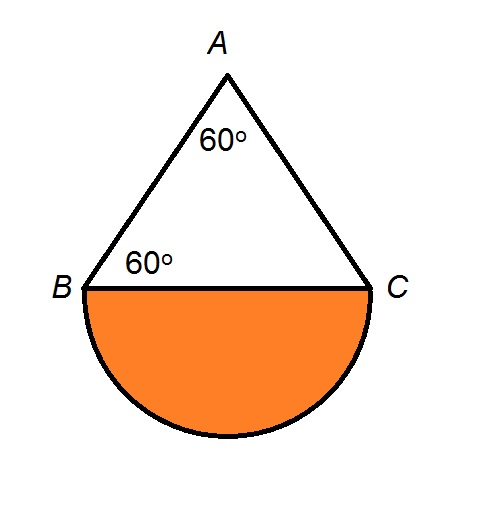Refer to the above figure, Which is the greater quantity?

(a) The area of the orange semicircle

(b) The area of(a) and (b) are equal

(b) is the greater quantity

It is impossible to determine which is greater from the information given

(a) is the greater quantity

(b) is the greater quantity

Explanation:has two angles of degree measure 60; its third angle must also have measure 60, makingan equilateral triangle

For the sake of simplicity, let; the reasoning is independent of the actual length. The area of equilateralcan be found by substituting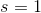in the formula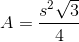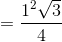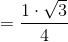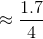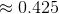Also, if, then the orange semicircle has diameter 1 and radius. Its area can be found by substitutingin the formula:has a greater area than the orange semicircle.

### Example Question #6 : Sectors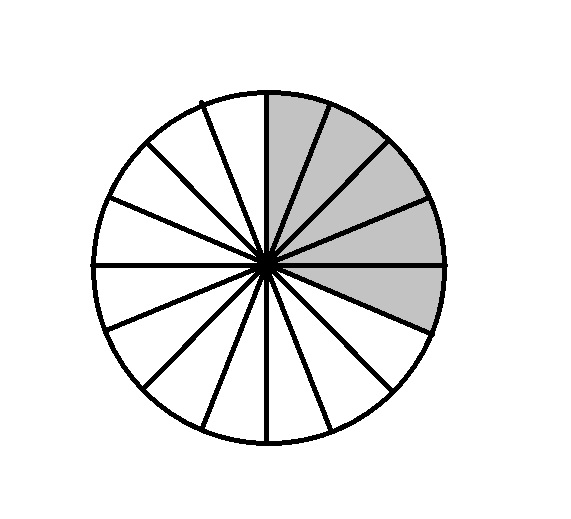The above circle, which is divided into sectors of equal size, has diameter 20. Give the area of the shaded region.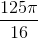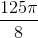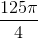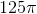Explanation:

The radius of a circle is half its diameter; the radius of the circle in the diagram is half of 20, or 10.

To find the area of the circle, set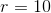in the area formula: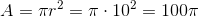The circle is divided into sixteen sectors of equal size, five of which are shaded; the shaded portion is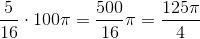.